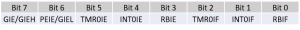A Timer is generally used for delay purposes or to trigger a particular event after a defined time interval as discussed in our previous chapter Timer and Counter with PIC18F4550. A timer used as an interrupt enhances this feature by freeing precious processor time used to poll for the timer overflow flag. Here we will learn how to set up a timer interrupt to trigger an event at a specific time interval.

## Timer Interrupt Configuration

Before setting up the timer, we have to enable the interrupt with the INTCON register as seen in our previous chapter Interrupt Handling in PIC18F4550.

### INTCON• GIE: Global Interrupt Enable bit. Enables all interrupts when set
• TMR0IE: TMR0 Overflow Interrupt Enable bit. Enables the TMR0 overflow interrupt when set
• TMR0IF: TMR0 Overflow Interrupt Flag bit. Sets when TMR0 register has overflowed

Since we will be using the Timer 0 for the interrupt, we have to enable the Global Interrupt Enable (GIE) bit and the Timer 0 Overflow Interrupt Enable (TMR0IE) bit.

Firmware Example:

```GIE = 1;
TMR0IE = 1;```

To configure timer interval, we have to specify the prescale and preload value while enabling Timer 0 with the T0CON and TMR0 registers as seen in our previous chapter Timer Delay Implementation in PIC18F4550. Here we will be setting up the interrupt to repeat every second.

Since the time interval required is large, we will use the prescale value as 256. To calculate the preload value for a 1-second delay at 20Mhz we use the equation,

Where,

• T – is the delay time in seconds
• Fosc – is the oscillator frequency (20 MHz)
• Prescale – is the maximum prescale value ( T0CON<2:0> )
• Resolution – is the maximum value of the timer ( 0xFFFF H )
• TMR0 – is the timer preload value ( TMR0H, TMR0L )

Thus the preload value, TMR0 can be calculated as

```TMR0 = Resolution - (( T * Fosc ) / (4 * Prescale))
= 65535 - ((1 * 20000000) / (4 * 256))
= 65535 - 19531
= 46004
= 0xB3B4 H```

Thus the prescale and preload value should be loaded as we designed.

Firmware Example:

```TMR0 = 0xB3B4;
T0CON = 0X87;```

## Interrupt Service Routine

The Interrupt Service Routine(ISR) or Interrupt Vector is a code sequence that is executed when an interrupt occurs. Here we require the ISR to execute every second. Inside the ISR we will be toggling the RB0 bit for demonstration purpose. After completing the purpose of the interrupt, we need to clear the interrupt flag T0IF in software and load the preload value to the TMR0 register to be ready for the next interrupt.

Firmware Example:

```__interrupt() void ISR(void)
{
if(T0IF)
{
LATB0=~LATB0;
TMR0 = 0xB3B4;
T0IF = 0;
}
}```